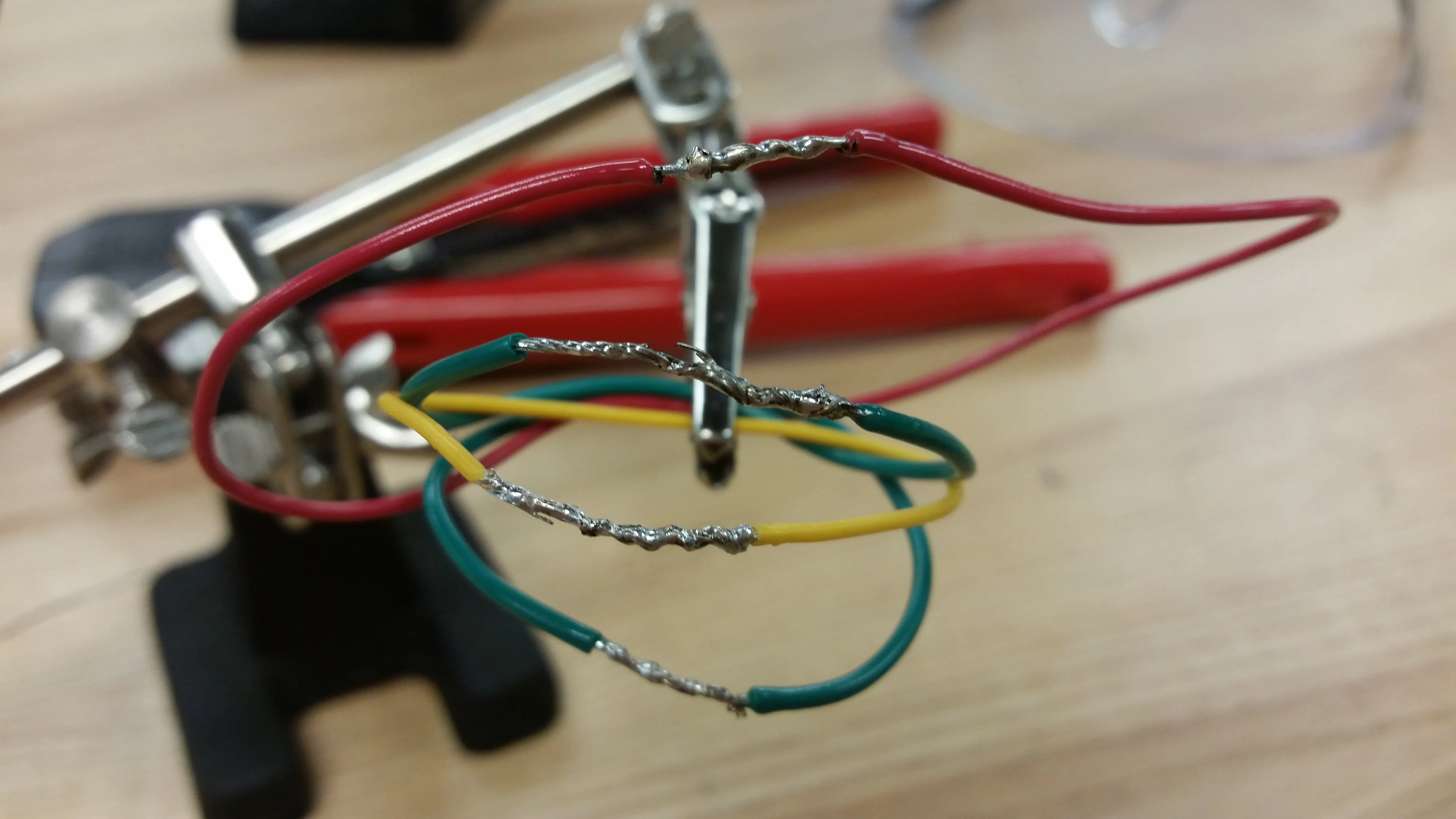Created October 1, 2015

# HW4: Soldering and Arduino Exercises

Soldering wires together, and Arduino exercises

Showcase (no instructions)52## Code

C/C++
Used different states (e.g. input is HIGH and led is OFF, or input is LOW and led is ON) to determine when the LED should blink and after what interval, without the delay function.
```const int ledPin =  13;   // initializing the variables that won't change
const int ledIn = 7;
int state = 0;   // 0 = LED off and 1 = LED on
char ledState;
unsigned long previousMillis = 0;        // will store last time LED was updated

const long interval = 500;
const long interval2 = 200; // interval at which to blink (milliseconds)

void setup() {
// set the digital pin as output:
pinMode(ledPin, OUTPUT);
pinMode(ledIn, INPUT);
}

void loop() { // The following is an unneccessarily robust program, and is where I have my problems
unsigned long currentMillis = millis();
if (ledState == LOW && state == 0){ // This is case #1, where the switch is not pressed and the led is off
if (currentMillis - previousMillis >= interval) {
// save the last time you blinked the LED
previousMillis = currentMillis;
}
digitalWrite(ledPin, HIGH); // Turn LED from off to on, then proceed to the following if statement
state = 1 - state;
}
else if (ledState == LOW && state == 1);{ // Still case #1, but now where the LED is on
if (currentMillis - previousMillis >= interval) {
// save the last time you blinked the LED
previousMillis = currentMillis;
}
digitalWrite(ledPin, LOW); // Turn LED off for time "interval", then proceed to the first if statment if ledState remains "LOW"
state = 1 - state;
}
if (ledState == HIGH && state == 0){ // This is case #2, where the switch is not pressed and the led is off
if (currentMillis - previousMillis >= interval2) {
// save the last time you blinked the LED
previousMillis = currentMillis;

digitalWrite(ledPin, HIGH);} // Turn the LED on now, then proceed to the following if statement
state = 1 - state;
}
else if (ledState == HIGH && state == 1);{ // Still case #2, but now where the LED is on
if (currentMillis - previousMillis >= interval2) {
// save the last time you blinked the LED
previousMillis = currentMillis;

digitalWrite(ledPin, LOW);} // Turn the LED off after time interval2, then the previous if statement will be activated if ledState remains "HIGH"
state = 1 - state;
}
}
```